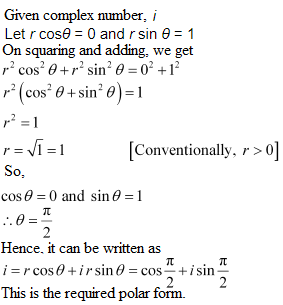# NCERT Solutions For Class 11 Math Chapter – 5 Exercise – 5.2

NCERT Solutions For Class 11 Math Chapter – 5 Exercise – 5.2

Find the modulus and the arguments of each of the complex numbers in Exercises 1 to 2.

1. z = – 1 – i √3

Solution: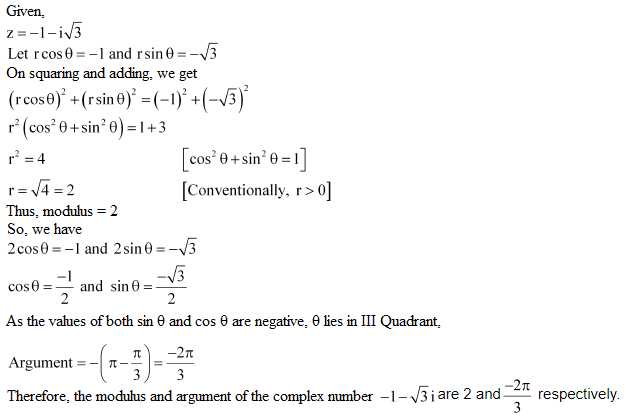2. z = -√3 + i

Solution: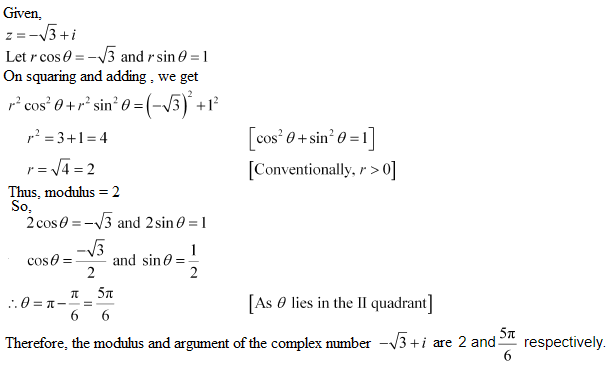Convert each of the complex numbers given in Exercises 3 to 8 in the polar form:

3. 1 – i

Solution:

class="" title="NCERT Solutions for Class 11 Chapter 5" src="https://cdn1.byjus.com/wp-content/uploads/2020/02/ncert-solutions-class-11-mathematics-chapter-5-ex-5-2-3.png" alt="NCERT Solutions Class 11 Mathematics Chapter 5 ex.5.2 - 3" />

4. – 1 + i

Solution: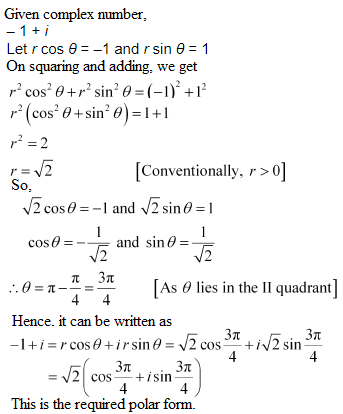5. – 1 – i

Solution: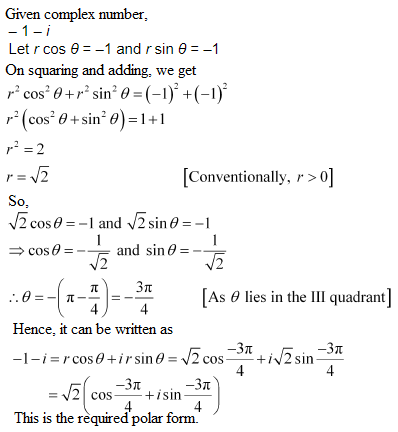6. – 3

Solution: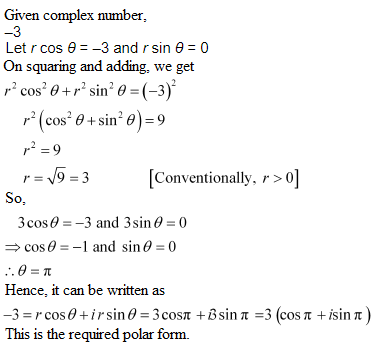7. 3 + i

Solution: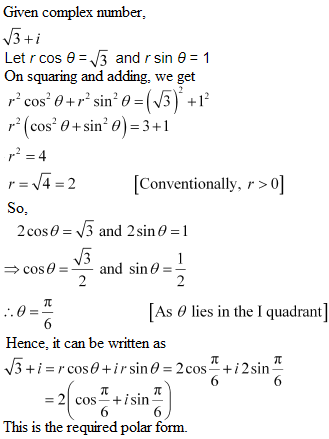8. i

Solution: